# regionprops

Measure properties of image regions

## Syntax

``stats = regionprops(BW,properties)``
``stats = regionprops(CC,properties)``
``stats = regionprops(L,properties)``
``stats = regionprops(___,I,properties)``
``stats = regionprops(output,___)``

## Description

example

````stats = regionprops(BW,properties)` returns measurements for the set of properties for each 8-connected component (object) in the binary image, `BW`. You can use `regionprops` on contiguous regions and discontiguous regions (see More About). NoteTo return measurements of a 3-D volumetric image, consider using `regionprops3`. While `regionprops` can accept 3-D images, `regionprops3` calculates more statistics for 3-D images than `regionprops`. For all syntaxes, if you do not specify the `properties` argument, then `regionprops` returns the `'Area'`, `'Centroid'`, and `'BoundingBox'` measurements.```
````stats = regionprops(CC,properties)` measures a set of properties for each connected component (object) in `CC`, which is a structure returned by `bwconncomp`.```
````stats = regionprops(L,properties)` measures a set of properties for each labeled region in label image `L`.```
````stats = regionprops(___,I,properties)` returns measurements for the set of properties specified by `properties` for each labeled region in the image `I`. The first input to `regionprops` (`BW`, `CC`, or `L`) identifies the regions in `I`.```

example

````stats = regionprops(output,___)` returns measurements for a set of properties, where `output` specifies the type of return value. `regionprops` can return measurements in a `struct` array or a `table`.```

## Examples

collapse all

Read a binary image into workspace.

`BW = imread('text.png');`

Calculate centroids for connected components in the image using `regionprops`. The `regionprops` function returns the centroids in a structure array.

`s = regionprops(BW,'centroid');`

Store the x- and y-coordinates of the centroids into a two-column matrix.

`centroids = cat(1,s.Centroid);`

Display the binary image with the centroid locations superimposed.

```imshow(BW) hold on plot(centroids(:,1),centroids(:,2),'b*') hold off```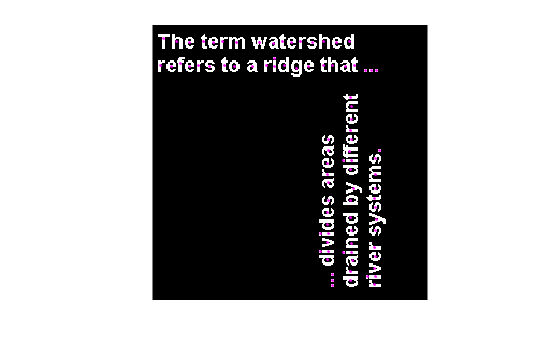Estimate the center and radii of circular objects in an image and use this information to plot circles on the image. In this example, `regionprops` returns the measured region properties in a table.

`a = imread('circlesBrightDark.png');`

Turn the input image into a binary image.

```bw = a < 100; imshow(bw) title('Image with Circles')```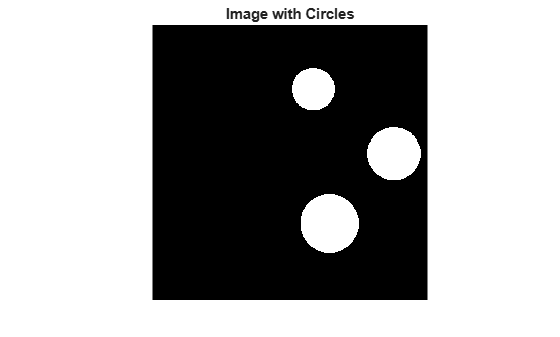Calculate properties of regions in the image and return the data in a table.

```stats = regionprops('table',bw,'Centroid',... 'MajorAxisLength','MinorAxisLength')```
```stats=4×3 table Centroid MajorAxisLength MinorAxisLength ________________ _______________ _______________ 256.5 256.5 834.46 834.46 300 120 81.759 81.759 330.47 369.83 111.78 110.36 450 240 101.72 101.72 ```

Get centers and radii of the circles.

```centers = stats.Centroid; diameters = mean([stats.MajorAxisLength stats.MinorAxisLength],2); radii = diameters/2;```

Plot the circles.

```hold on viscircles(centers,radii); hold off```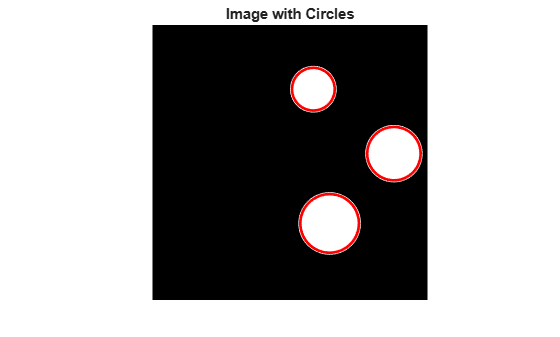## Input Arguments

collapse all

Binary image, specified as a logical array of any dimension.

Data Types: `logical`

Connected components, specified as a structure returned by `bwconncomp`.

Data Types: `struct`

Label image, specified as one of the following.

• A numeric array of any dimension. Pixels labeled `0` are the background. Pixels labeled `1` make up one object; pixels labeled `2` make up a second object; and so on. `regionprops` treats negative-valued pixels as background and rounds down input pixels that are not integers. You can get a numeric label image from labeling functions such as `watershed` or `labelmatrix`.

• A categorical array. Each category corresponds to a different region.

Data Types: `single` | `double` | `int8` | `int16` | `int32` | `uint8` | `uint16` | `uint32` | `categorical`

Type of measurement, specified as a comma-separated list of string scalars or character vectors, a cell array of string scalars or character vectors, or as `'all'` or `'basic'`.

• If you specify `'all'`, then `regionprops` computes all the shape measurements and, for grayscale images, the pixel value measurements as well.

• If you specify `'basic'`, then `regionprops` computes only the `'Area'`, `'Centroid'`, and `'BoundingBox'` measurements.

The following tables list all the properties that provide shape measurements. The properties listed in the Pixel Value Measurements table are valid only when you specify a grayscale image.

Shape Measurements

Property NameDescriptionN-D SupportGPU SupportCode Generation
`'Area'`

Actual number of pixels in the region, returned as a scalar. (This value might differ slightly from the value returned by `bwarea`, which weights different patterns of pixels differently.)

To find the equivalent to the area of a 3-D volume, use the `'Volume'` property of `regionprops3`.

YesYesYes
`'BoundingBox'`

Position and size of the smallest box containing the region, returned as a 1-by-(2*Q) vector. The first Q elements are the coordinates of the minimum corner of the box. The second Q elements are the size of the box along each dimension. For example, a 2-D bounding box with value `[5.5 8.5 11 14]` indicates that the (x,y) coordinate of the top-left corner of the box is (5.5, 8.5), the horizontal width of the box is 11 pixels, and the vertical height of the box is 14 pixels.

YesYesYes
`'Centroid'`

Center of mass of the region, returned as a 1-by-`Q` vector. The first element of `Centroid` is the horizontal coordinate (or x-coordinate) of the center of mass. The second element is the vertical coordinate (or y-coordinate). All other elements of `Centroid` are in order of dimension. This figure illustrates the centroid and bounding box for a discontiguous region. The region consists of the white pixels; the green box is the bounding box, and the red dot is the centroid.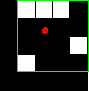YesYesYes
`'Circularity'`

Roundness of objects, returned as a struct with field `Circularity`. The struct contains the circularity value for each object in the input image. The circularity value is computed as `(4*Area*pi)/(Perimeter2)`. For a perfect circle, the circularity value is 1. The input must be a label matrix or binary image with contiguous regions. If the image contains discontiguous regions, `regionprops` returns unexpected results.

Note

`Circularity` is not recommended for very small objects such as a 3-by-3 square. For such cases, the results might exceed the circularity value for a perfect circle.

2-D onlyNoYes
`'ConvexArea'`Number of pixels in `'ConvexImage'`, returned as a scalar.2-D onlyNoNo
`'ConvexHull'`Smallest convex polygon that can contain the region, returned as a p-by-2 matrix. Each row of the matrix contains the x- and y-coordinates of one vertex of the polygon.2-D onlyNoNo
`'ConvexImage'`Image that specifies the convex hull, with all pixels within the hull filled in (set to `on`), returned as a binary image (`logical`). The image is the size of the bounding box of the region. (For pixels that the boundary of the hull passes through, `regionprops` uses the same logic as `roipoly` to determine whether the pixel is inside or outside the hull.) 2-D onlyNoNo
`'Eccentricity'`Eccentricity of the ellipse that has the same second-moments as the region, returned as a scalar. The eccentricity is the ratio of the distance between the foci of the ellipse and its major axis length. The value is between 0 and 1. (0 and 1 are degenerate cases. An ellipse whose eccentricity is 0 is actually a circle, while an ellipse whose eccentricity is 1 is a line segment.)2-D onlyYesYes
`'EquivDiameter'`Diameter of a circle with the same area as the region, returned as a scalar. Computed as `sqrt(4*Area/pi)`.2-D onlyYesYes
`'EulerNumber'`Number of objects in the region minus the number of holes in those objects, returned as a scalar. This property is supported only for 2-D label matrices. `regionprops` uses 8-connectivity to compute the Euler number (also known as the Euler characteristic). To learn more about connectivity, see Pixel Connectivity.2-D onlyNoYes
`'Extent'`Ratio of pixels in the region to pixels in the total bounding box, returned as a scalar. Computed as the `Area` divided by the area of the bounding box.2-D onlyYesYes
`'Extrema'`

Extrema points in the region, returned as an 8-by-2 matrix. Each row of the matrix contains the x- and y-coordinates of one of the points. The format of the vector is ```[top-left top-right right-top right-bottom bottom-right bottom-left left-bottom left-top]```. This figure illustrates the extrema of two different regions. In the region on the left, each extrema point is distinct. In the region on the right, certain extrema points (such as `top-left` and `left-top`) are identical.2-D onlyYesYes
`'FilledArea'`Number of `on` pixels in `FilledImage`, returned as a scalar.YesNoYes
`'FilledImage'`

Image the same size as the bounding box of the region, returned as a binary (`logical`) array. The `on` pixels correspond to the region, with all holes filled in, as shown in this figure.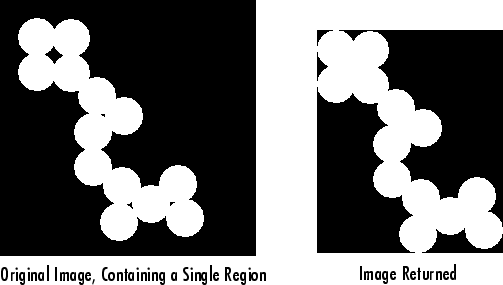YesNoYes
`'Image'`Image the same size as the bounding box of the region, returned as a binary (`logical`) array. The `on` pixels correspond to the region, and all other pixels are `off`.YesYesYes
`'MajorAxisLength'`Length (in pixels) of the major axis of the ellipse that has the same normalized second central moments as the region, returned as a scalar.2-D onlyYesYes
`'MaxFeretProperties'`

Feret properties that include maximum Feret diameter, its relative angle, and coordinate values, returned as a struct with fields:

 Field Description `MaxFeretDiameter` Maximum Feret diameter measured as the maximum distance between any two boundary points on the antipodal vertices of convex hull that enclose the object. `MaxFeretAngle` Angle of the maximum Feret diameter with respect to horizontal axis of the image. `MaxFeretCoordinates` Endpoint coordinates of the maximum Feret diameter.

The input can be a binary image, connected component, or a label matrix.

2-D onlyNoNo
`'MinFeretProperties'`

Feret properties that include minimum Feret diameter, its relative angle, and coordinate values, returned as a struct with fields:

 Field Description `MinFeretDiameter` Minimum Feret diameter measured as the minimum distance between any two boundary points on the antipodal vertices of convex hull that enclose the object. `MinFeretAngle` Angle of the minimum Feret diameter with respect to horizontal axis of the image. `MinFeretCoordinates` Endpoint coordinates of the minimum Feret diameter.

The input can be a binary image, a connected component, or a label matrix.

2-D onlyNoNo
`'MinorAxisLength'`Length (in pixels) of the minor axis of the ellipse that has the same normalized second central moments as the region, returned as a scalar.2-D onlyYesYes
`'Orientation'`

Angle between the x-axis and the major axis of the ellipse that has the same second-moments as the region, returned as a scalar. The value is in degrees, ranging from -90 degrees to 90 degrees. This figure illustrates the axes and orientation of the ellipse. The left side of the figure shows an image region and its corresponding ellipse. The right side shows the same ellipse with the solid blue lines representing the axes. The red dots are the foci. The orientation is the angle between the horizontal dotted line and the major axis.2-D onlyYesYes
`'Perimeter'`

Distance around the boundary of the region returned as a scalar. `regionprops` computes the perimeter by calculating the distance between each adjoining pair of pixels around the border of the region. If the image contains discontiguous regions, `regionprops` returns unexpected results. This figure illustrates the pixels included in the perimeter calculation for this object.2-D onlyNoYes
`'PixelIdxList'`Linear indices of the pixels in the region, returned as a p-element vector. YesYesYes
`'PixelList'`Locations of pixels in the region, returned as a p-by-`Q` matrix. Each row of the matrix has the form `[x y z ...]` and specifies the coordinates of one pixel in the region.YesYesYes
`'Solidity'`Proportion of the pixels in the convex hull that are also in the region, returned as a scalar. Computed as `Area/ConvexArea`.2-D onlyNoNo
`'SubarrayIdx'`Elements of `L` inside the object bounding box, returned as a cell array that contains indices such that `L(idx{:})` extracts the elements.YesYesNo

The pixel value measurement properties in the following table are valid only when you specify a grayscale image, `I`.

Pixel Value Measurements

Property Name DescriptionN-D SupportGPU SupportCode Generation
`'MaxIntensity'`Value of the pixel with the greatest intensity in the region, returned as a scalar.YesYesYes
`'MeanIntensity'`Mean of all the intensity values in the region, returned as a scalar.YesYesYes
`'MinIntensity'`Value of the pixel with the lowest intensity in the region, returned as a scalar.YesYesYes
`'PixelValues'`Number of pixels in the region, returned as a p-by-1 vector, where p is the number of pixels in the region. Each element in the vector contains the value of a pixel in the region.YesYesYes
`'WeightedCentroid'`Center of the region based on location and intensity value, returned as a p-by-`Q` vector of coordinates. The first element of `WeightedCentroid` is the horizontal coordinate (or x-coordinate) of the weighted centroid. The second element is the vertical coordinate (or y-coordinate). All other elements of `WeightedCentroid` are in order of dimension. YesYesYes

Data Types: `char` | `string` | `cell`

Image to be measured, specified as a grayscale image. The size of the image must match the size of the binary image `BW`, connected component structure `CC`, or label image `L`.

Data Types: `single` | `double` | `int8` | `int16` | `int32` | `int64` | `uint8` | `uint16` | `uint32`

Return type, specified as either of the following values.

ValueDescription
`'struct'`Returns an array of structures with length equal to the number of objects in `BW`, `CC.NumObjects`, or `max(L(:))`. The fields of the structure array denote different properties for each region, as specified by `properties`.
`'table'`

Returns a `table` with height (number of rows) equal to the number of objects in `BW`, `CC.NumObjects`, or `max(L(:))`. The variables (columns) denote different properties for each region, as specified by `properties`.

Data Types: `char` | `string`

## Output Arguments

collapse all

Measurement values, returned as an array of structs or a table. The number of structs in the array or the number of rows in the table is equal to the number of objects in `BW`, `CC.NumObjects`, or `max(L(:))`. The fields of each struct or the variables in each row denote the properties calculated for each region, as specified by `properties`. If the input image is a categorical label image `L`, then `stats` includes an additional field or variable with the property `'LabelName'`.

collapse all

### Contiguous Regions and Discontiguous Regions

Contiguous regions are also called objects, connected components, or blobs. A label image `L` containing contiguous regions might look like this:

```1 1 0 2 2 0 3 3 1 1 0 2 2 0 3 3```

Elements of `L` equal to 1 belong to the first contiguous region or connected component; elements of `L` equal to 2 belong to the second connected component; and so on.

Discontiguous regions are regions that can contain multiple connected components. A label image containing discontiguous regions might look like this:

```1 1 0 1 1 0 2 2 1 1 0 1 1 0 2 2```
Elements of `L` equal to 1 belong to the first region, which is discontiguous and contains two connected components. Elements of `L` equal to 2 belong to the second region, which is a single connected component.

## Tips

• The `ismember` function is useful for creating a binary image containing only objects or regions that meet certain criteria. For example, these commands create a binary image containing only the regions whose area is greater than 80 and whose eccentricity is less than 0.8.

```cc = bwconncomp(BW); stats = regionprops(cc,'Area','Eccentricity'); idx = find([stats.Area] > 80 & [stats.Eccentricity] < 0.8); BW2 = ismember(labelmatrix(cc),idx); ```
• The default connectivity is 8-connected for 2-D images, and maximal connectivity for higher dimensions. To specify nondefault connectivity, use `bwconncomp` to create the connected components and then pass the result to `regionprops`.

• `regionprops` takes advantage of intermediate results when computing related measurements. Therefore, it is fastest to compute all the desired measurements in a single call to `regionprops`.

• Most of the measurements take little time to compute. However, these measurements can take longer, depending on the number of regions in `L`:

• `'ConvexHull'`

• `'ConvexImage'`

• `'ConvexArea'`

• `'FilledImage'`